## 女子举重问题

article2023/12/11 13:33:52/

# 一、问题的描述

1、搜集各个级别世界女子举重比赛的实际数据。分别建立女子举重比赛总成绩的线性模型、幂函数模型、幂函数改进模型，并最终建立总冠军评选模型。

2、请对以上模型作进一步的改进，或提出更好的模型，并应用模型进行排名。

3、同样对最近举行的一届奥运会所有男女举重冠军行进评选，选出1名最优秀的举重运动员，给出你的定量评选方法并合理论述。

4、由以上研究过程及结果结论的启发，你认为这个模型可以推广应用到生活或学习中的哪些情况下，或者应该对生活或学习中哪些事物现有的处理方式需要做出改进，请给出你的分析。

# 二、搜集资料和数据

1.级别的划分

（男子举重原有8个级别是：56、62、69、77、85、94、105公斤级和105公斤以上级，于2018年由国际举联调整新的10个级别是55（非奥）、61、67、73、81、89（非奥）、96、102（非奥）、109公斤级和109公斤以上级共10个级别。女子举重原来7个级别是：48、53、58、63、69、75公斤级和75公斤级以上级，新的10个级别也调整为：45（非奥）、49、55、59、64、71（非奥）、76、81（非奥）、87公斤级和87公斤以上级。男女6个非奥级别只在奥运会以外的赛事举行。）

2.搜集数据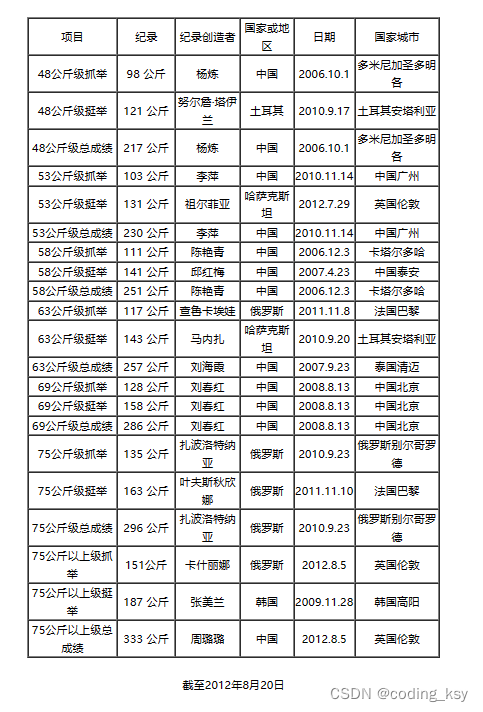在这个网站里面，我们需要选取，当前记录这一栏目，千万千万千万记住，不要用旧纪录这一栏，因为在2018年之后男子和女子的分类的级别已经改变。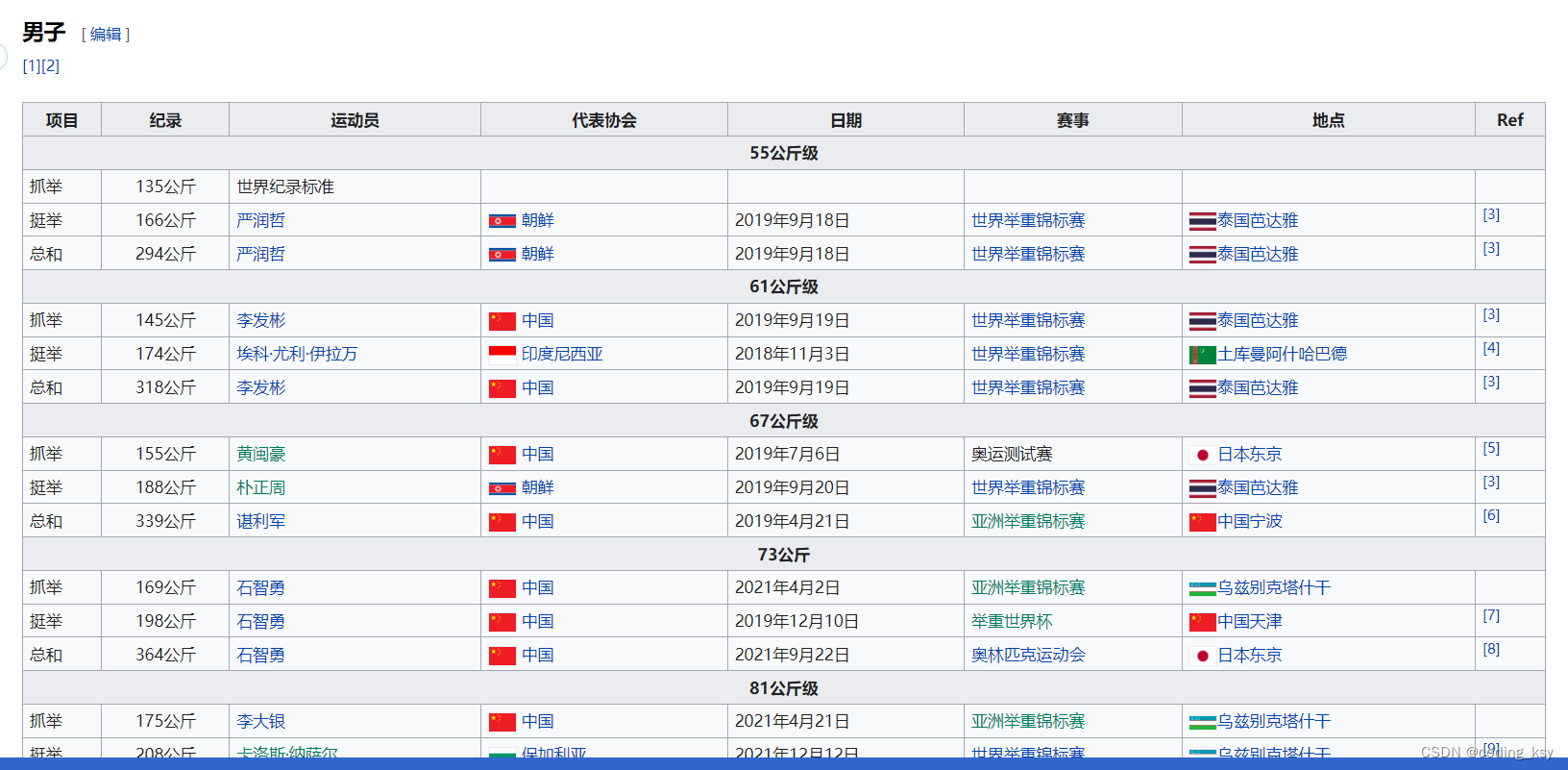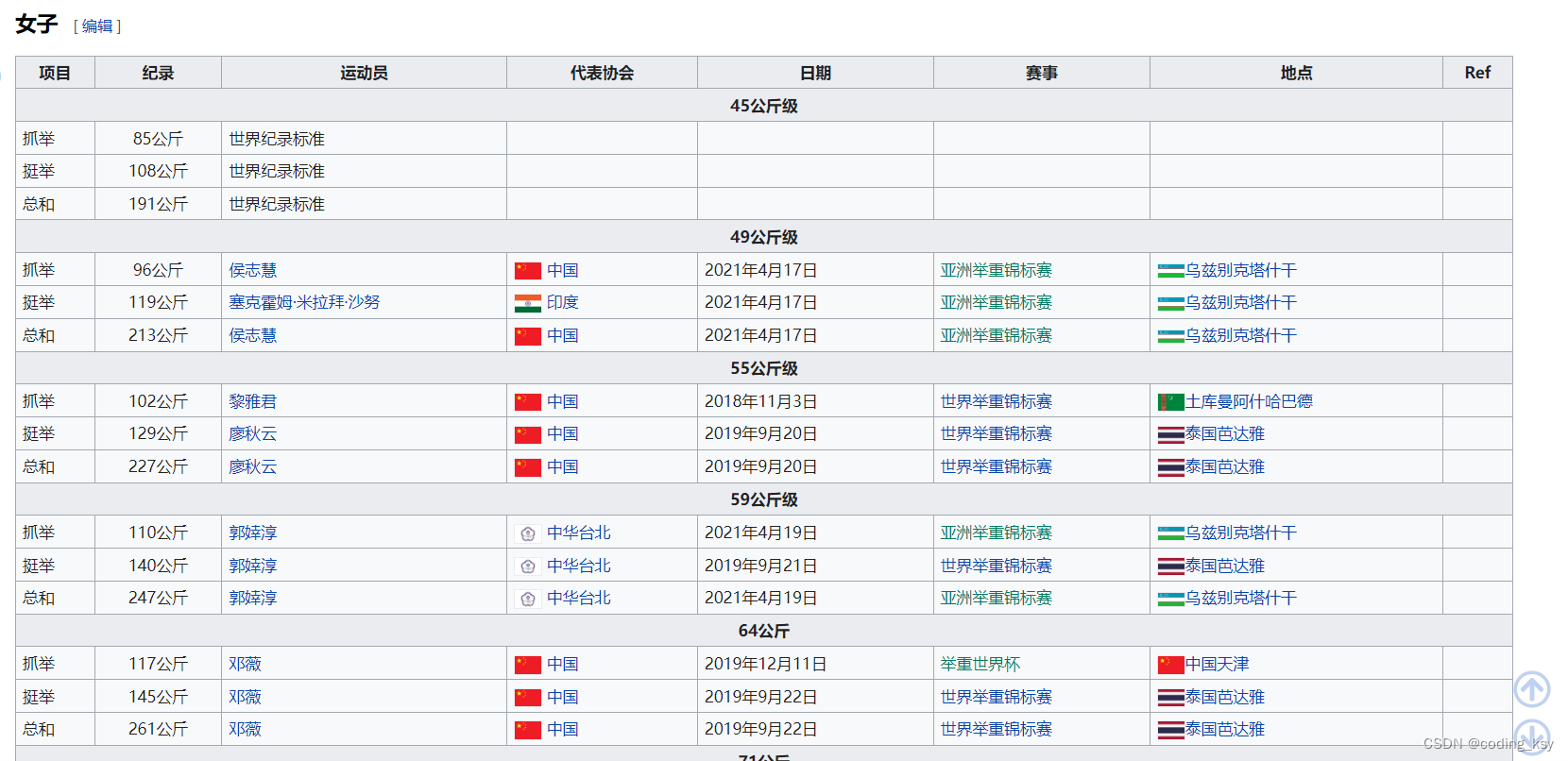图3.女子新纪录

# 三、第一问

## 1.分别绘制抓举、挺举、总和下的散点图如下：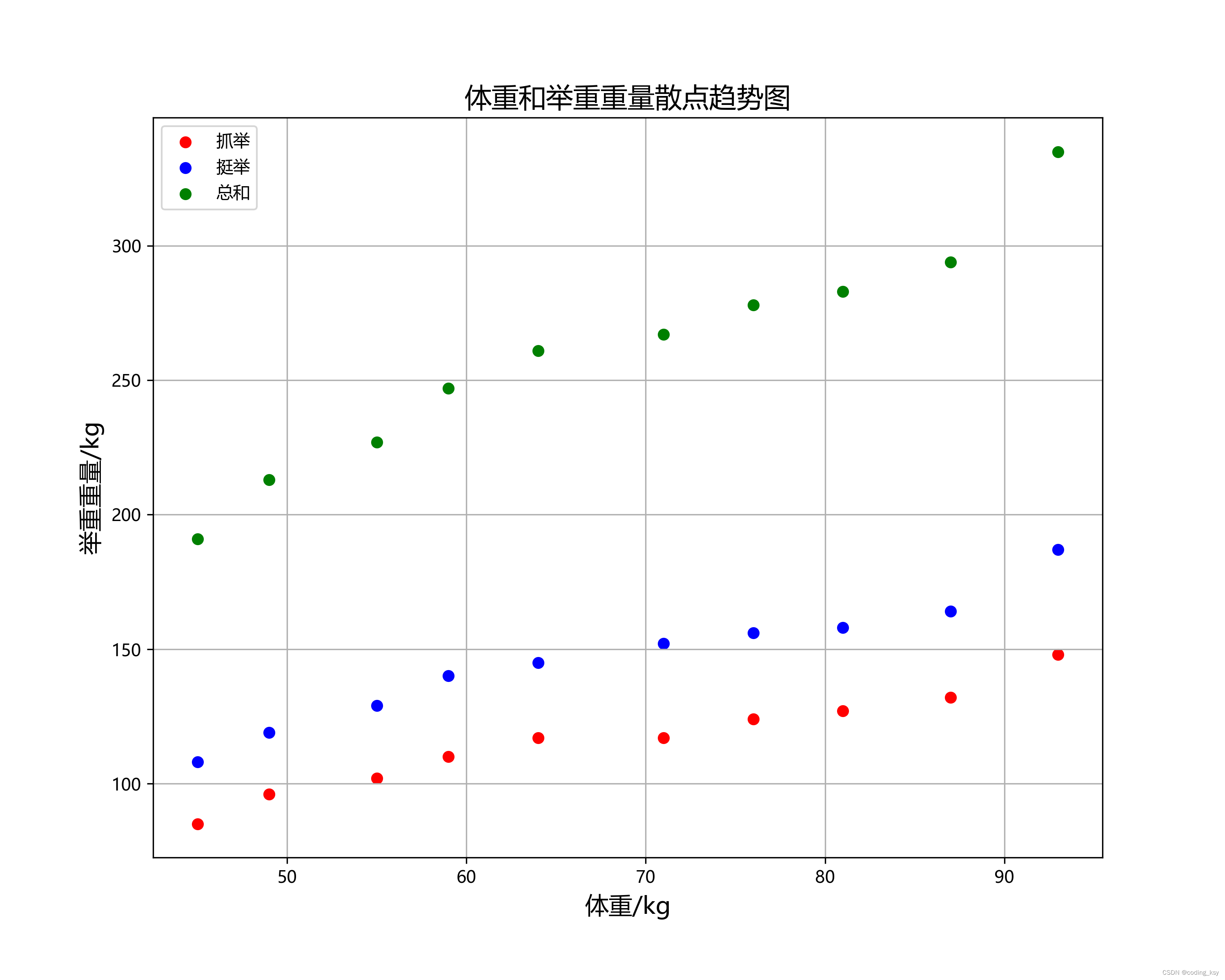分析图形可能为线性关系、非线性关系（指数、对数等）。

``````#引入相应的库
import numpy as np
import pandas as pd
import matplotlib.pyplot as plt
import seaborn as sns

#读取数据
#选取抓举、挺举、总和
data_1=data[data["class"]=="抓举"].sort_values("weight")
data_2=data[data["class"]=="挺举"].sort_values("weight")
data_3=data[data["class"]=="总和"].sort_values("weight")
#筛选出自变量和因变量
x1=data_1["weight"].values
y1=data_1["record"].values
x2=data_2["weight"].values
y2=data_2["record"].values
x3=data_3["weight"].values
y3=data_3["record"].values

#解决中文乱码和符号显示的问题
plt.rcParams['font.sans-serif']=['SimHei']
plt.rcParams['font.sans-serif']=['Microsoft YaHei']
#绘制散点图
plt.figure(figsize=(10,8),dpi=300)
plt.title("体重和举重重量散点趋势图",fontsize="16")
plt.xlabel("体重/kg",fontsize="14")
plt.ylabel("举重重量/kg",fontsize="14")
plt.scatter(x1,y1,color="red",label="抓举")
plt.scatter(x2,y2,color="blue",label="挺举")
plt.scatter(x3,y3,color="green",label="总和")
plt.grid(True)
plt.legend()
plt.savefig("1.png")``````

## 2.线性拟合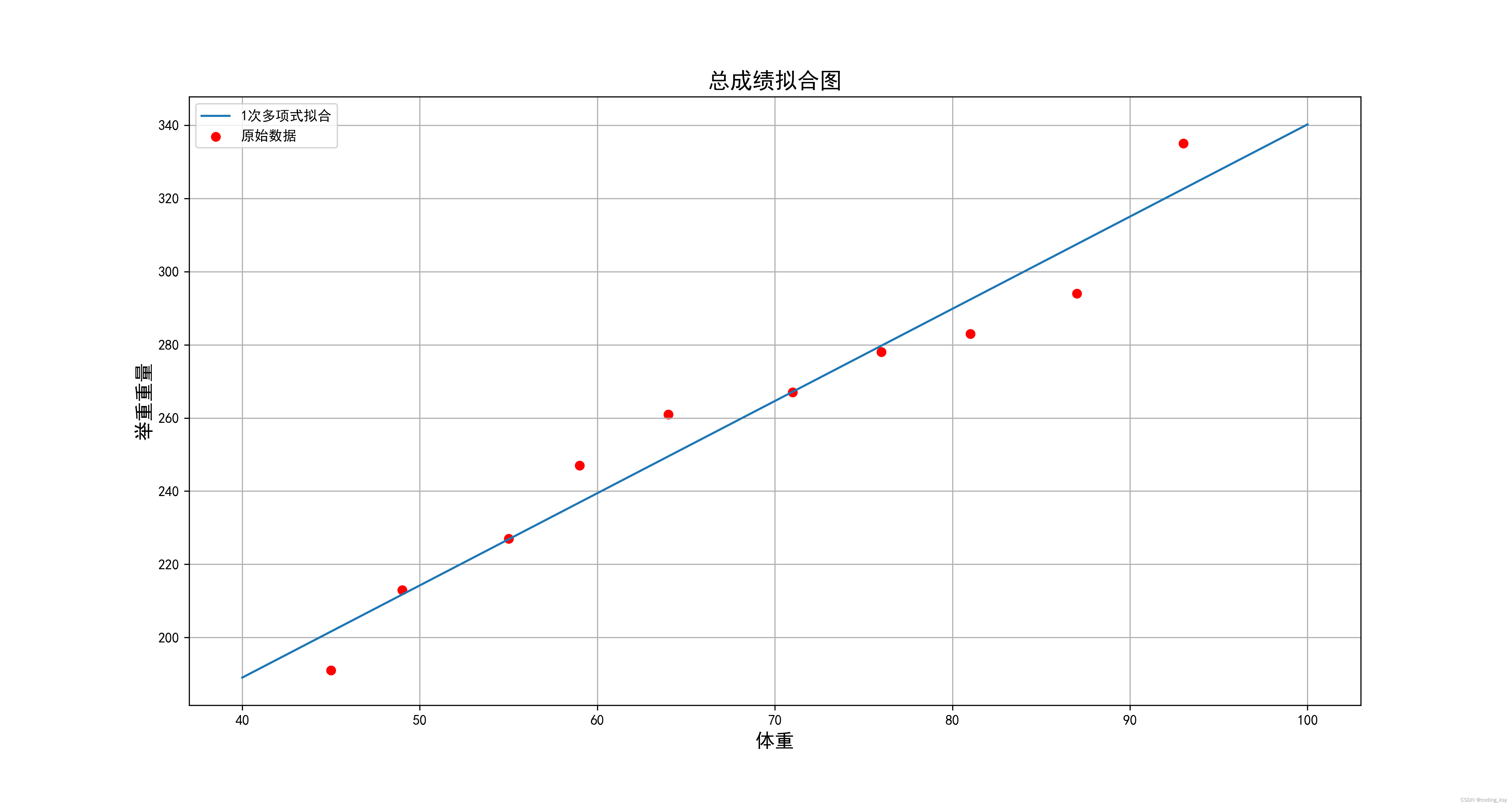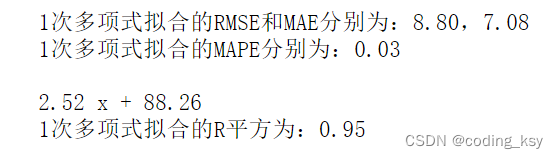## 2.幂函数拟合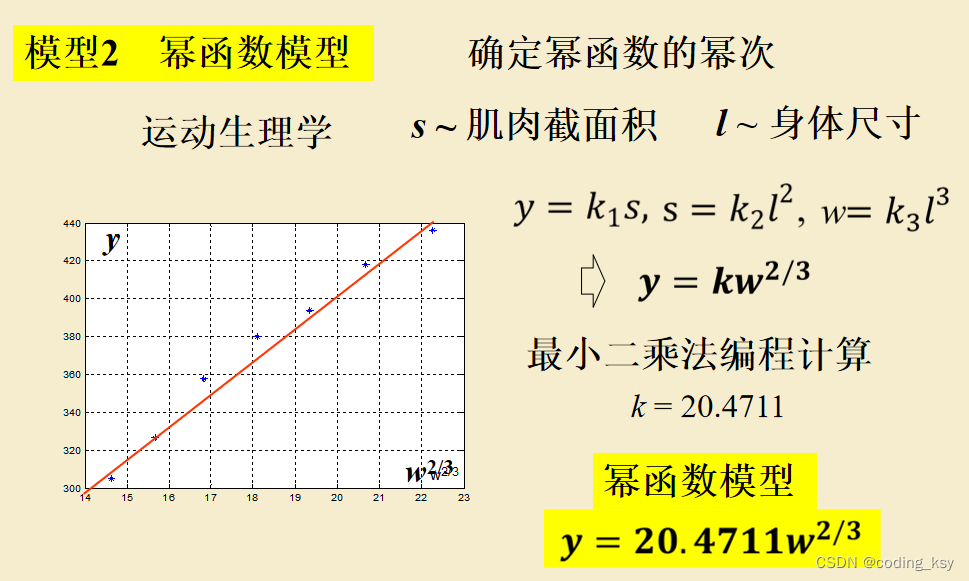上图中的参数是举例子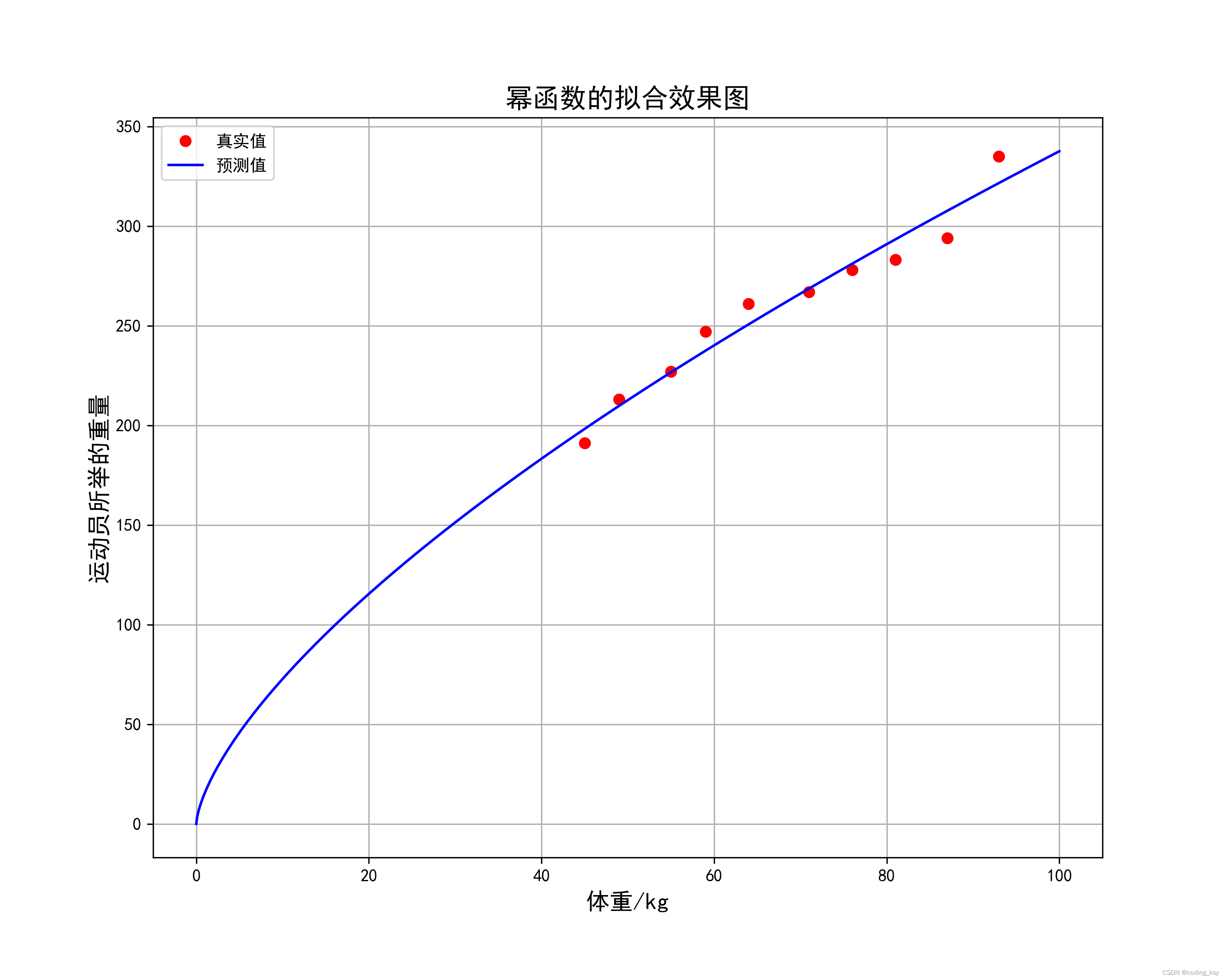模型的评估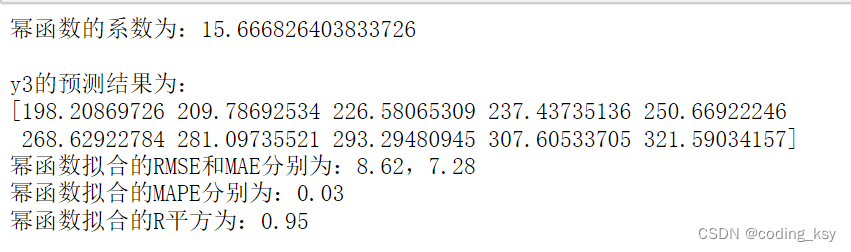上面的参数RMSE和MAE来计算误差的话，无法排除离群点的影响，所以采用了MAPE来计算误差的话，这样就排除离群点对模型的影响。

``````#幂函数进行拟合

#引入相应的库
import numpy as np
import matplotlib.pyplot as plt
from scipy.optimize import curve_fit

# 自定义的拟合函数
def func2(x,k):
return k*np.power(x,2/3)

# 开始拟合
popt, pcov = curve_fit(func2, x3, y3)

# 提取拟合结果
k = popt#幂函数的系数
print("幂函数的系数为：{:}\n".format(k))
print("y3的预测结果为：")
y_predict=func2(x3,k)#预测的结果值
print(y_predict)

x_new = np.linspace(0, 100, 1000) # 生成新的x轴数据
#绘制拟合曲线效果
plt.figure(figsize=(10,8),dpi=300)
plt.title("幂函数的拟合效果图",fontsize=16)
plt.xlabel("体重/kg",fontsize=14)
plt.ylabel("运动员所举的重量",fontsize=14)
plt.plot(x3,y3,"ro",label="真实值")
plt.plot(x_new,func2(x_new,k),color='blue',label="预测值")
plt.grid(True)
plt.legend(loc="upper left")
plt.savefig("3.png")

#误差分析
rmse_poly = np.sqrt(np.mean((y3 - func2(x3,k))**2))
mae_poly = np.mean(np.abs(y3 - func2(x3,k)))
mape_poly=np.mean(np.abs(y3-func2(x3,k))/func2(x3,k))

# 打印RMSE和MAE
print("幂函数拟合的RMSE和MAE分别为：{:.2f}，{:.2f}".format(rmse_poly, mae_poly))
print("幂函数拟合的MAPE分别为：{:.2f}".format(mape_poly))
y_poly = poly(x_new)
# 计算R平方
r2_poly = r2_score(y3, func2(x3,k))
# 打印R平方
print("幂函数拟合的R平方为：{:.2f}".format(r2_poly))
print("\n")``````

## 3.改进幂函数的拟合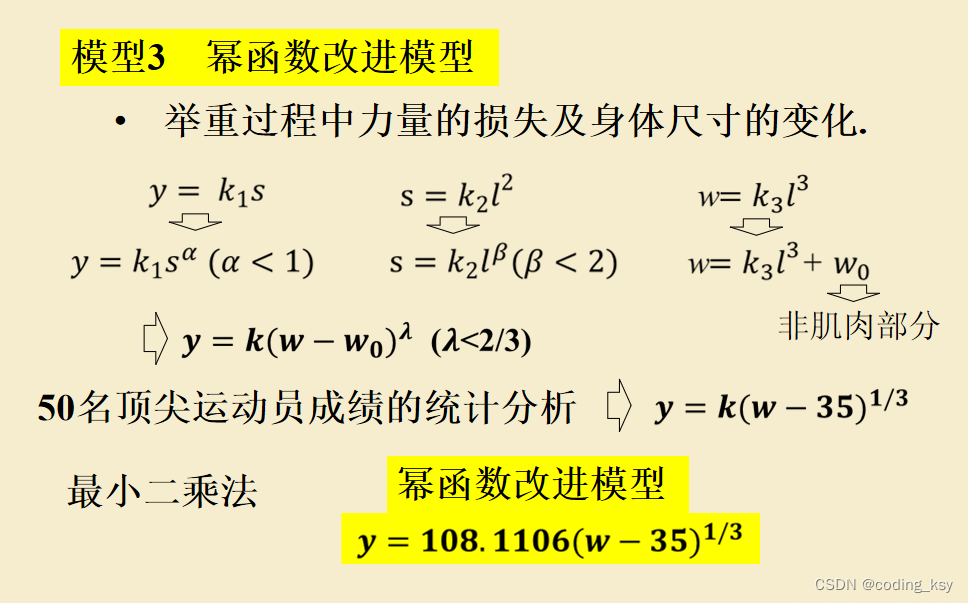上图中的参数是举例子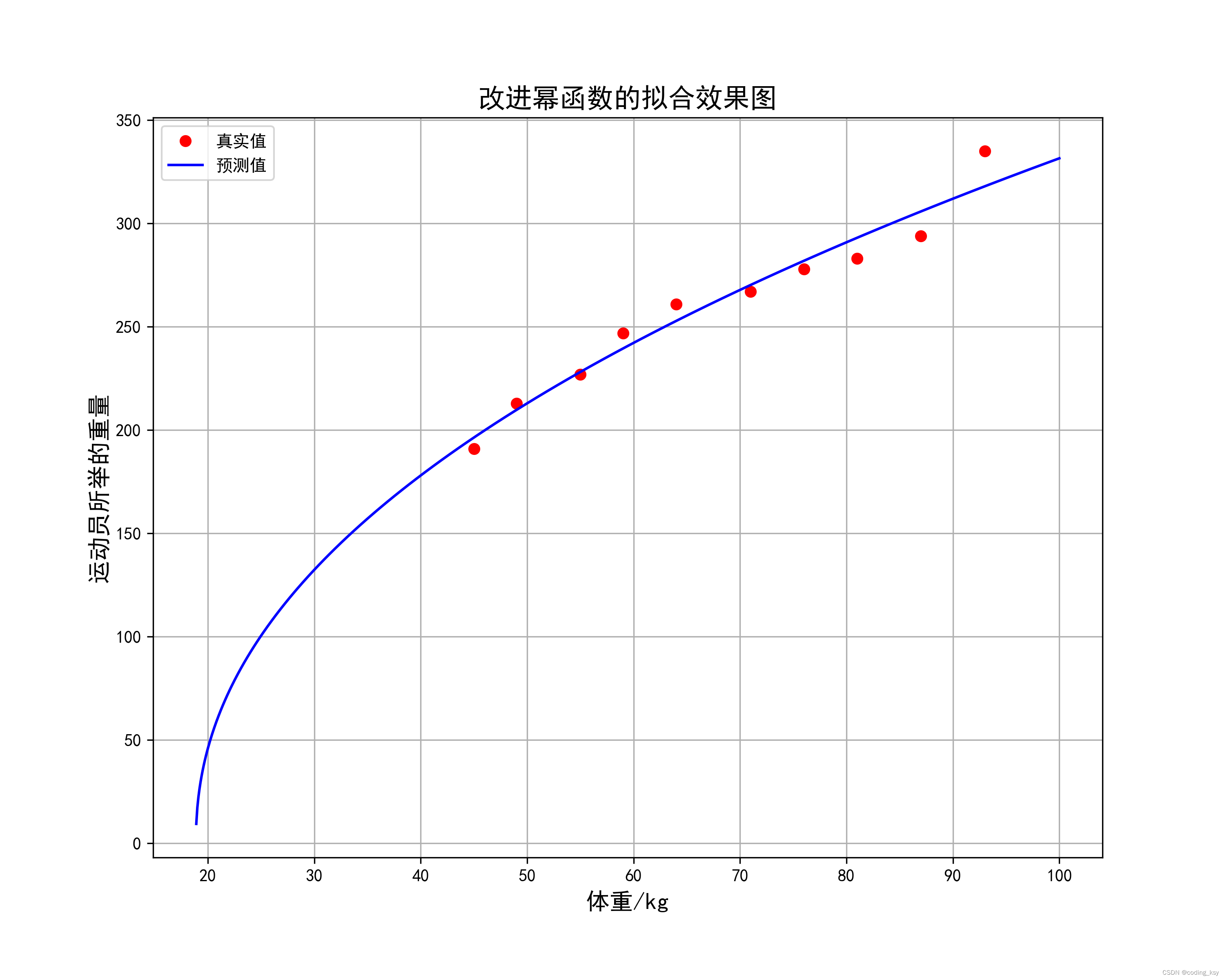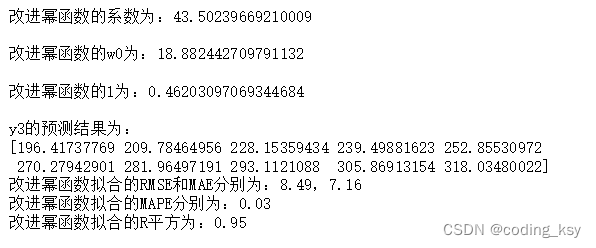上面的参数RMSE和MAE来计算误差的话，无法排除离群点的影响，所以采用了MAPE来计算误差的话，这样就排除离群点对模型的影响。

``````#改进幂函数
#幂函数进行拟合

#引入相应的库
import numpy as np
import matplotlib.pyplot as plt
from scipy.optimize import curve_fit

# 自定义的拟合函数
def func2(x,k,w0,l):
return k*np.power(x-w0,l)

# 开始拟合
popt, pcov = curve_fit(func2, x3, y3)

# 提取拟合结果
k = popt#改进幂函数的系数k
w0=popt#改进幂函数的w0
l=popt#改进幂函数的l
print("改进幂函数的系数为：{:}\n".format(k))
print("改进幂函数的w0为：{:}\n".format(w0))
print("改进幂函数的l为：{:}\n".format(l))
print("y3的预测结果为：")
y_predict=func2(x3,k,w0,l)#预测的结果值
print(y_predict)

x_new = np.linspace(0, 100, 1000) # 生成新的x轴数据
#绘制拟合曲线效果
plt.figure(figsize=(10,8),dpi=300)
plt.title("改进幂函数的拟合效果图",fontsize=16)
plt.xlabel("体重/kg",fontsize=14)
plt.ylabel("运动员所举的重量",fontsize=14)
plt.plot(x3,y3,"ro",label="真实值")
plt.plot(x_new,func2(x_new,k,w0,l),color='blue',label="预测值")
plt.grid(True)
plt.legend(loc="upper left")
plt.savefig("4.png")

#误差分析
rmse_poly = np.sqrt(np.mean((y3 - func2(x3,k,w0,l))**2))
mae_poly = np.mean(np.abs(y3 - func2(x3,k,w0,l)))
mape_poly=np.mean(np.abs(y3-func2(x3,k,w0,l))/func2(x3,k,w0,l))

# 打印RMSE和MAE
print("改进幂函数拟合的RMSE和MAE分别为：{:.2f}，{:.2f}".format(rmse_poly, mae_poly))
print("改进幂函数拟合的MAPE分别为：{:.2f}".format(mape_poly))
y_poly = poly(x_new)
# 计算R平方
r2_poly = r2_score(y3, func2(x3,k,w0,l))
# 打印R平方
print("改进幂函数拟合的R平方为：{:.2f}".format(r2_poly))
print("\n")``````

综上所述，线性模型（一次）、幂函数模型、改进幂函数模型的效果大致相同，因此可以选择其中一个模型来进行下面的操作，为保持与二题的连贯性，建议选择线性模型，其次是改进幂函数模型，最后为幂函数模型。

## 4.标准统一化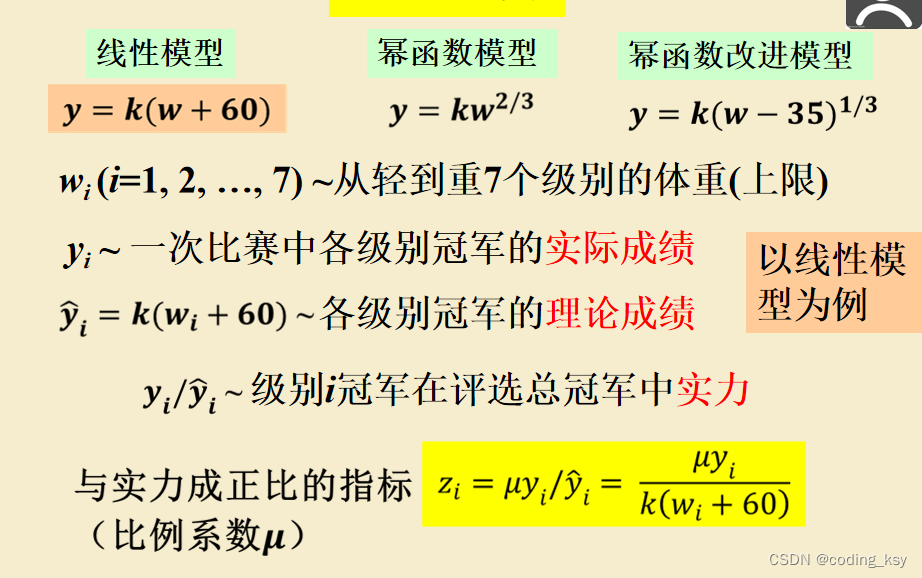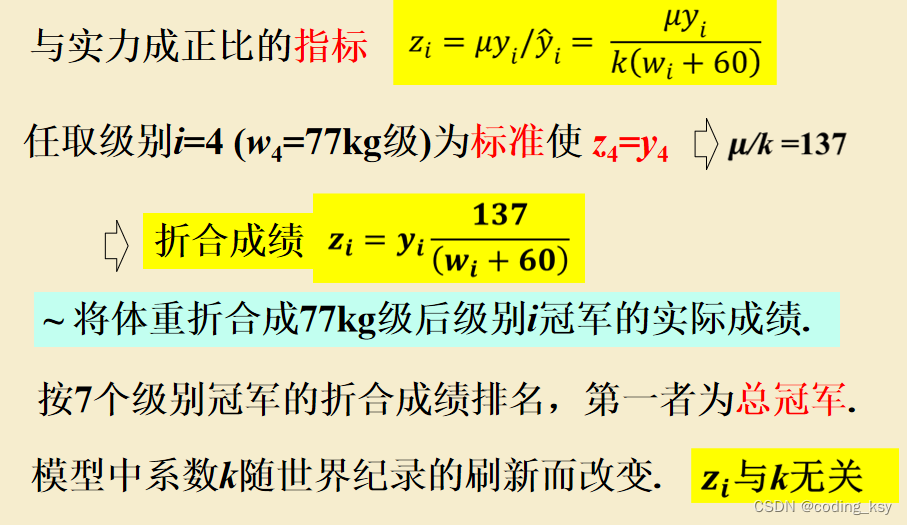本文选取中间级别71公斤的作为标准，使所以级别的数据转换到71公斤的标准下。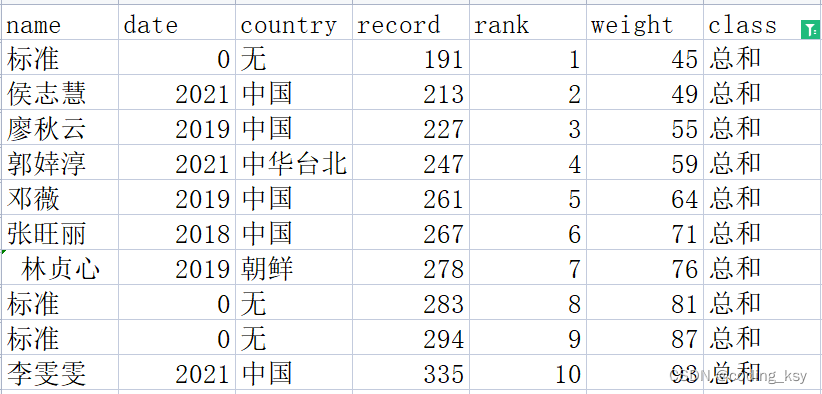选用线性模型（一次），当然也可以选择其它模型（改进幂函数等）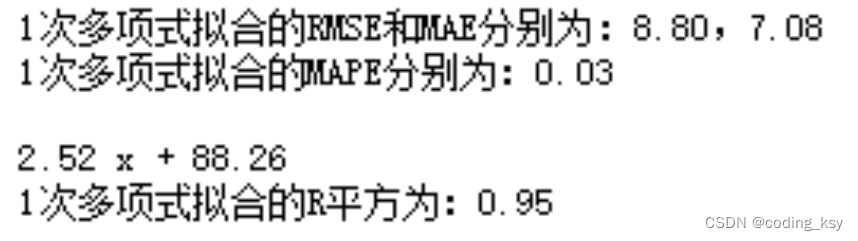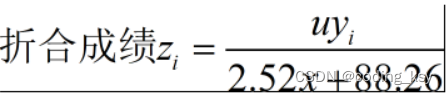将级别6的数据代入，即可算出u=267.18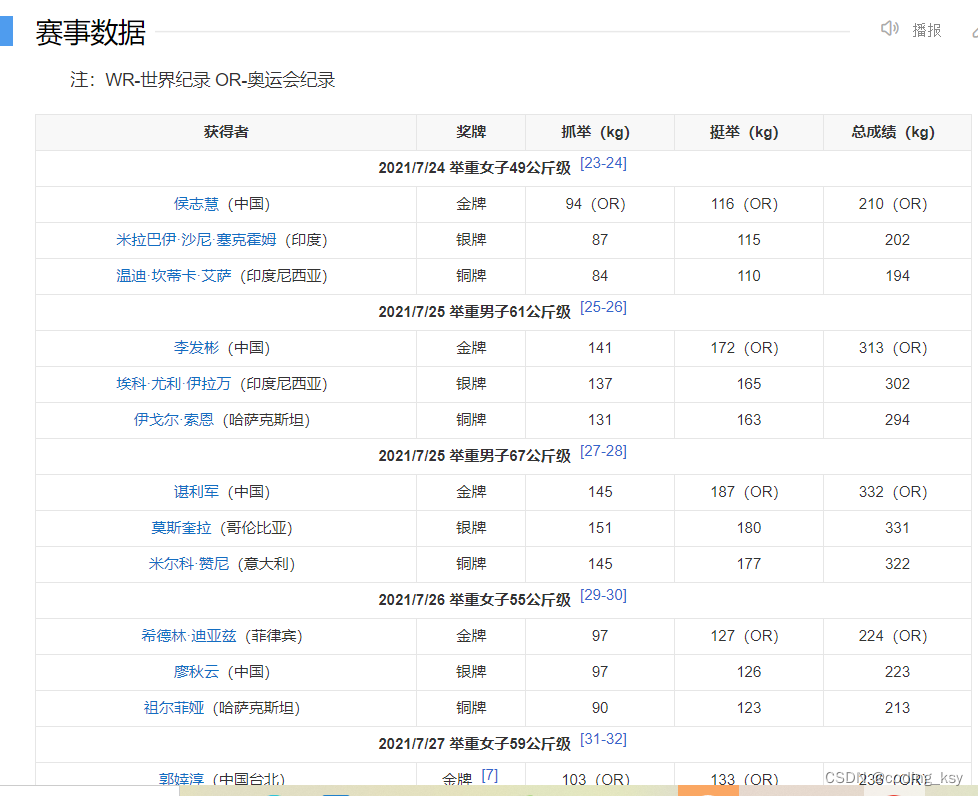数据处理：在当年东京奥运会有的级别中选取总冠军，以该级别的最高边界值作为选手的体重。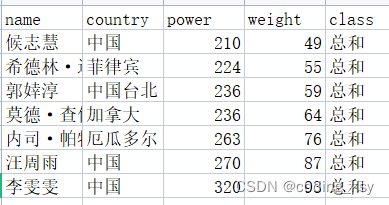计算得出得结果如下：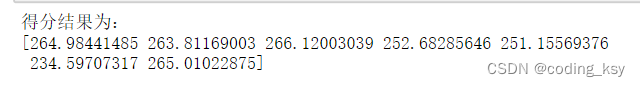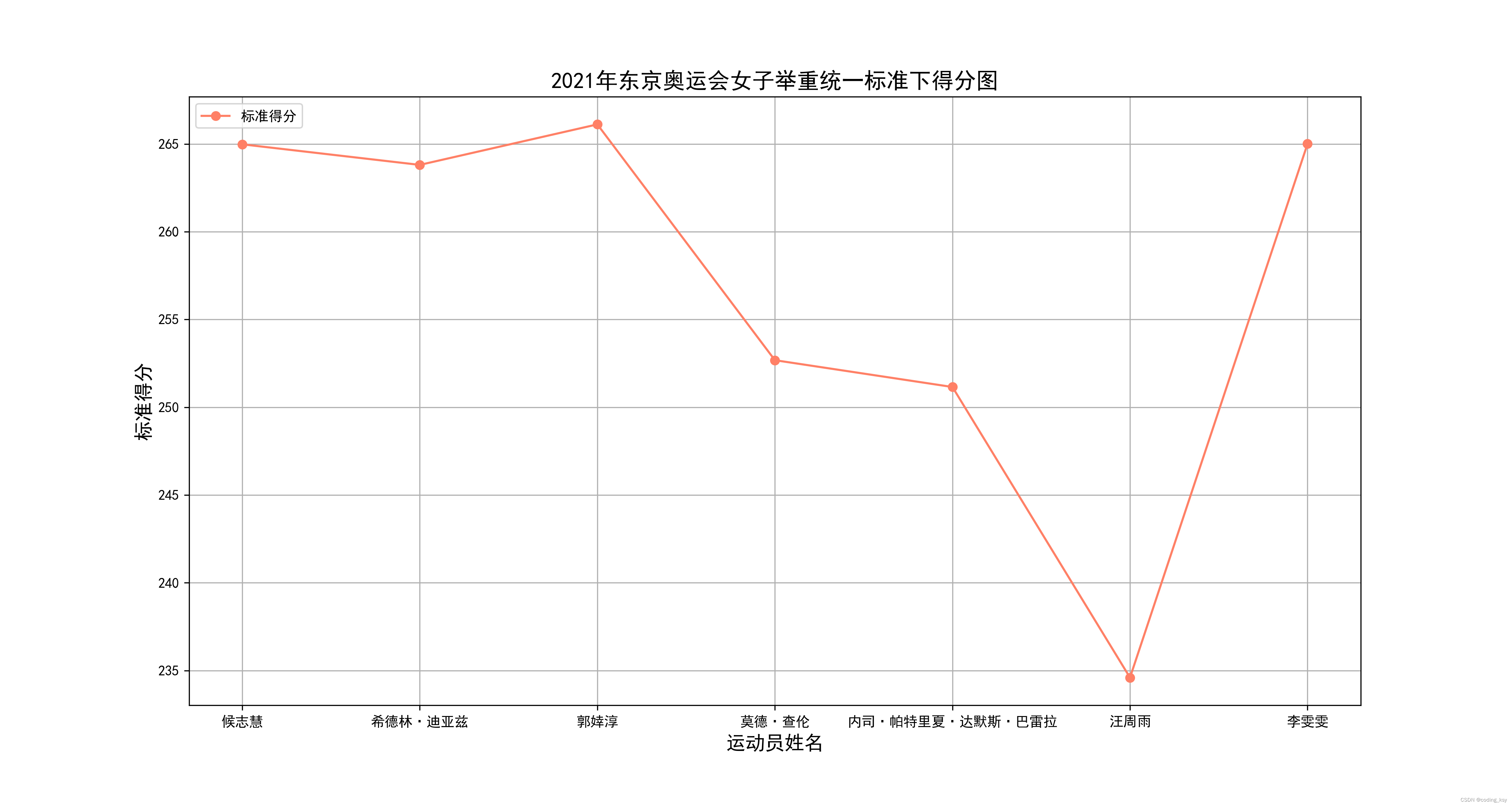从上图可知：中国台北的郭婞淳为总冠军

``````#导库
import numpy as np
import pandas as pd
import matplotlib.pyplot as plt
#读取数据
x4=data["weight"].values
y4=data["power"].values
#定义标准化函数
coefficients = np.polyfit(x3, y3, 1) # 用1次多项式进行拟合
poly = np.poly1d(coefficients) # 生成多项式函数
def zfunc(x,y):
return 267.18*y/(2.52*x+88.26)

#求解运动员标准得分，该分数越大，证明运动员成绩越好
z=zfunc(x4,y4)
#将数据读入到data中
data["标准化得分"]=z

#画图
x5=data["name"].values
y5=z
plt.figure(figsize=(15,8),dpi=300)
plt.title("2021年东京奥运会女子举重统一标准下得分图",fontsize=16)
plt.xlabel("运动员姓名",fontsize=14)
plt.ylabel("标准得分",fontsize=14)
plt.plot(x5,y5,color="#FF8066",marker="o",label="标准得分")
plt.grid(True)
plt.legend(loc="upper left")
plt.savefig("5.png")
print("得分结果为：")
print(z)``````

# 三、第二问（多项式拟合）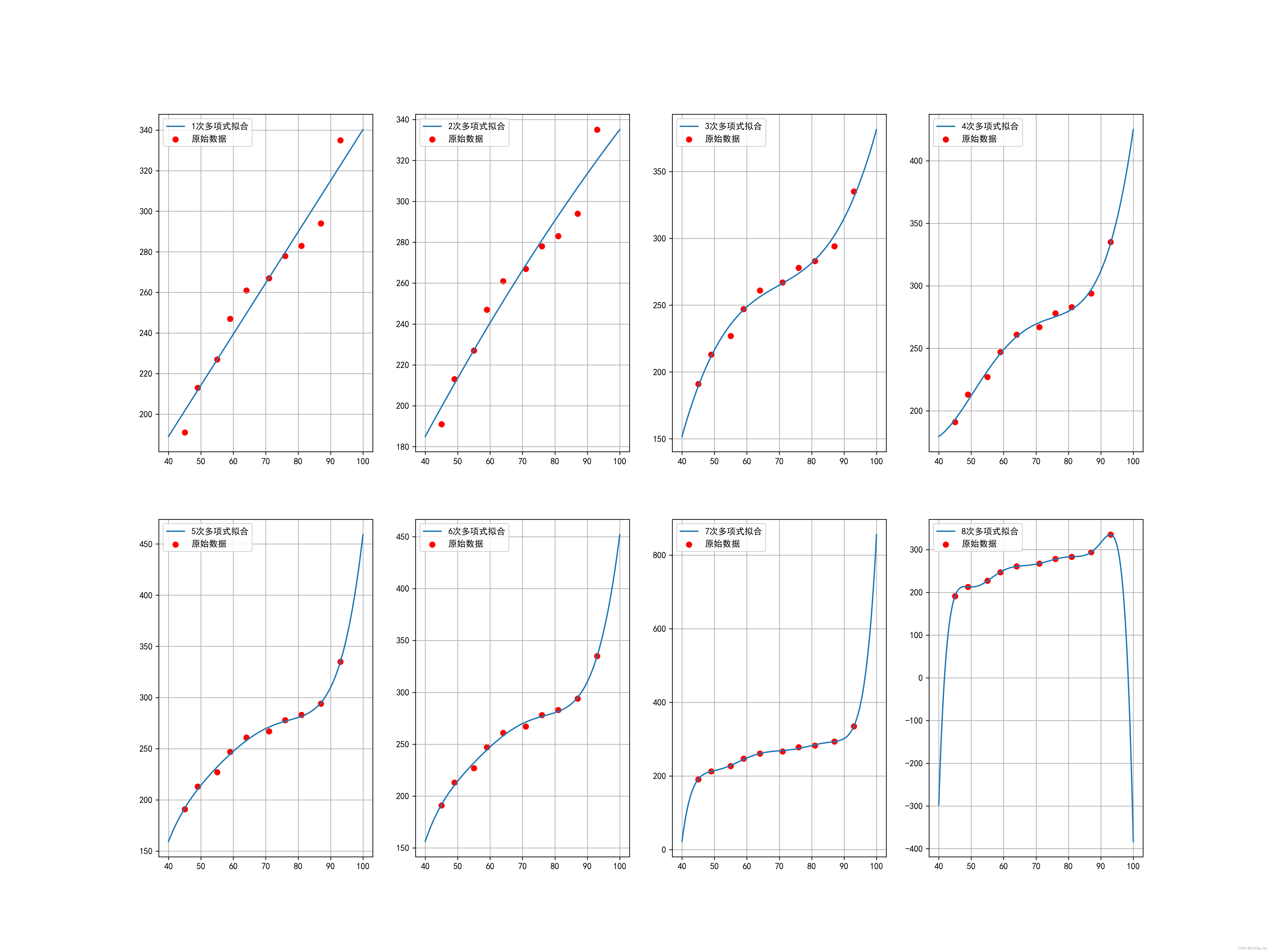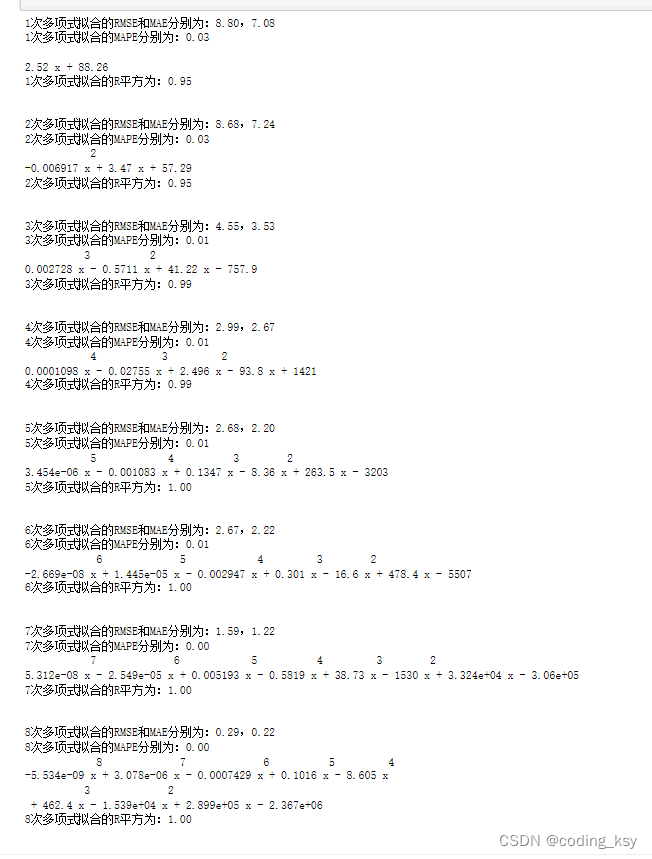``````from scipy.interpolate import interp1d
import numpy as np
import matplotlib.pyplot as plt
from sklearn.metrics import r2_score

plt.rcParams['font.sans-serif']=['SimHei'] #用来正常显示中文标签
plt.rcParams['axes.unicode_minus'] = False #用来正常显示负号
plt.figure(figsize=(20,15),dpi=300)
plt.title("总成绩拟合图",fontsize=16)
plt.xlabel("体重",fontsize=14)
plt.ylabel("举重重量",fontsize=14)
for i in range(1,9):
x_new = np.linspace(40, 100, 1000) # 生成新的x轴数据
coefficients = np.polyfit(x3, y3, i) # 用5次多项式进行拟合
poly = np.poly1d(coefficients) # 生成多项式函数
# 绘图
plt.subplot(2,4,i)
plt.grid(True)
plt.scatter(x3,y3,marker="o",color="red", label='原始数据')
plt.plot(x_new, poly(x_new), label=str(i)+'次多项式拟合')
plt.legend(loc="upper left")
plt.savefig("2.png")``````
``````# 计算拟合后的数值
for i in range(1,9):
coefficients = np.polyfit(x3, y3, i) # 用5次多项式进行拟合
poly = np.poly1d(coefficients) # 生成多项式函数
y_poly = poly(x_new)
# # 计算RMSE和MAE
# rmse_cubic = np.sqrt(np.mean((y - f_cubic(x))**2))
# mae_cubic = np.mean(np.abs(y - f_cubic(x)))
rmse_poly = np.sqrt(np.mean((y3 - poly(x3))**2))
mae_poly = np.mean(np.abs(y3 - poly(x3)))
mape_poly=np.mean(np.abs(y3-poly(x3))/poly(x3))

# 打印RMSE和MAE
print(str(i)+"次多项式拟合的RMSE和MAE分别为：{:.2f}，{:.2f}".format(rmse_poly, mae_poly))
print(str(i)+"次多项式拟合的MAPE分别为：{:.2f}".format(mape_poly))
print(poly)
y_poly = poly(x_new)
# 计算R平方
r2_poly = r2_score(y3, poly(x3))
# 打印R平方
print(str(i)+"次多项式拟合的R平方为：{:.2f}".format(r2_poly))
print("\n")``````

从上面的结果中我们可以看出，当多项式的最高次数大于4次的时后，虽然模型的R的平方较好，但是不符合实际情况，属于过拟合现象，上面的参数RMSE和MAE来计算误差的话，无法排除离群点的影响，所以采用了MAPE来计算误差的话，这样就排除离群点对模型的影响。这个多项式拟合可以用到第二问当中去，作为模型的改进，因此我们进行的优化为多项式优化。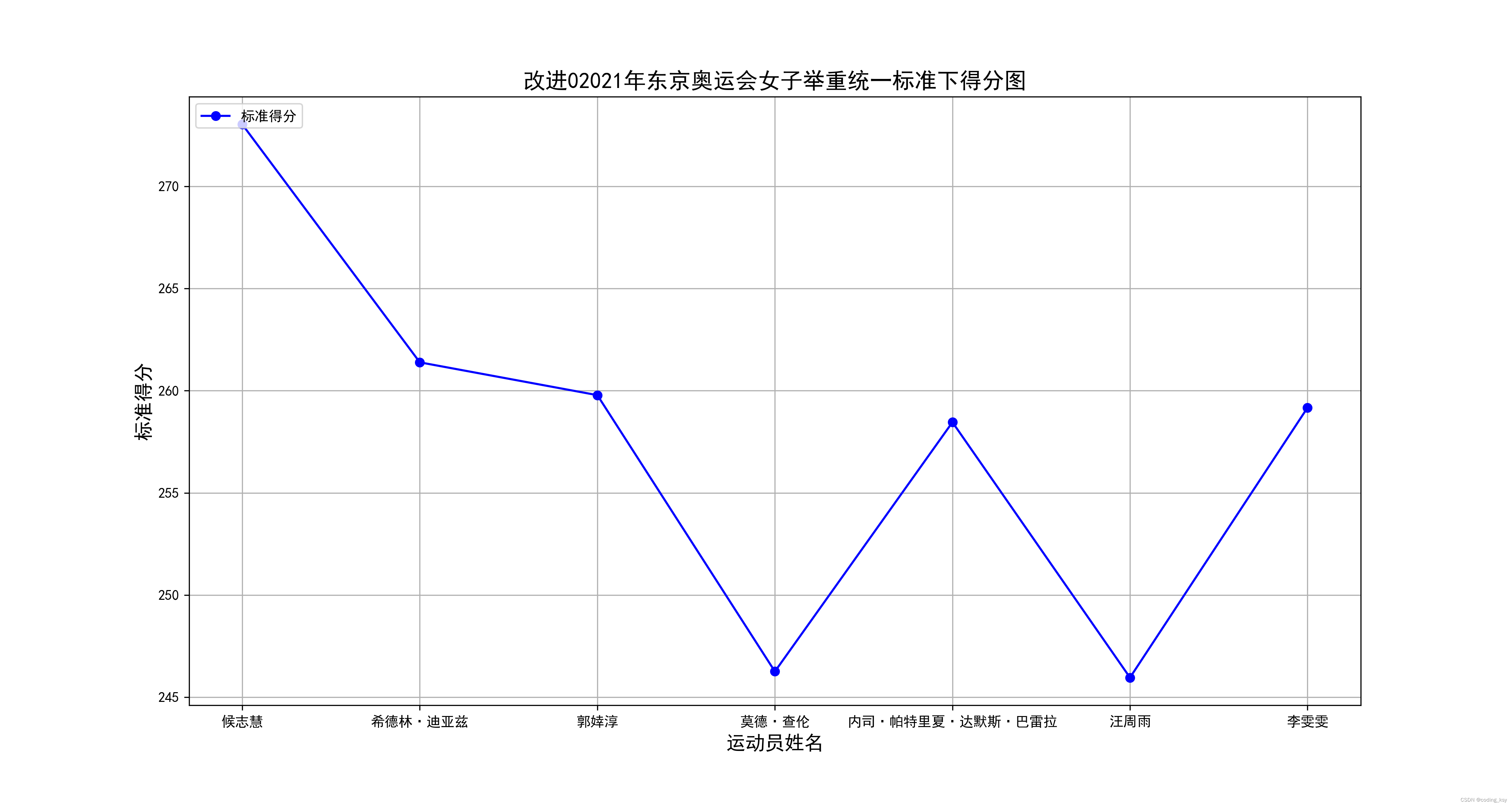从图中可以清晰的看出：在4次多项式的条件下候志惠的标准的分最高。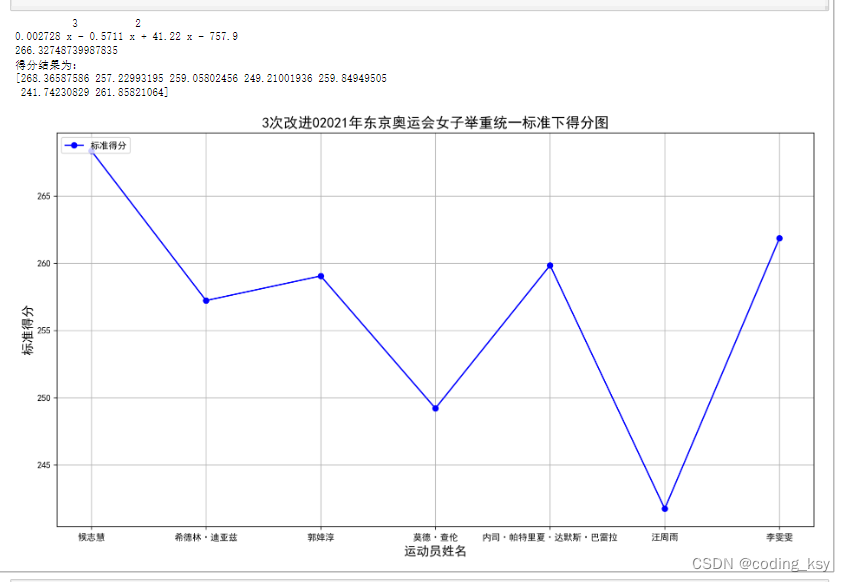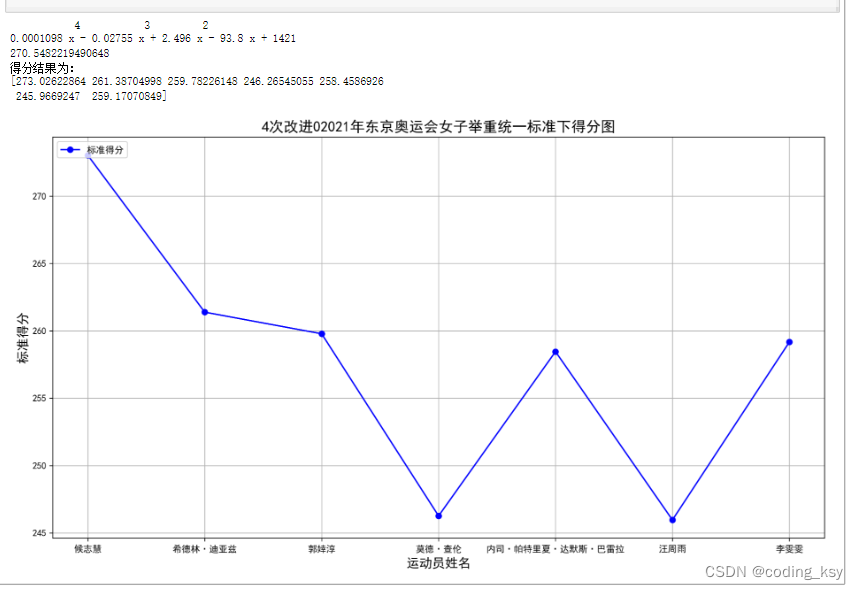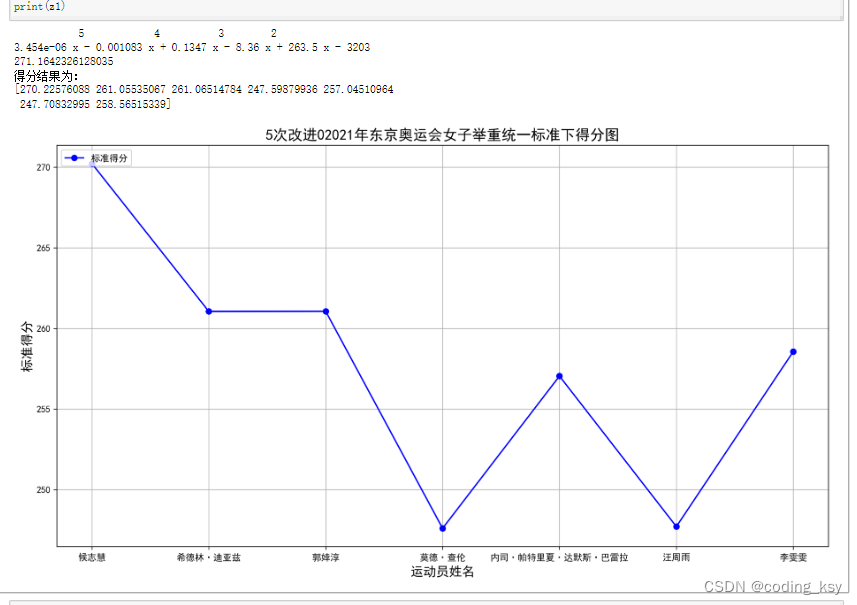在这就不再一一列举了，从上面可以观察出随着最高次数的增加，模型逐渐趋于稳定。

``````#导库
import numpy as np
import pandas as pd
import matplotlib.pyplot as plt

#读取数据
x4=data["weight"].values
y4=data["power"].values

#改进的模型，4次多项式拟合
coefficients = np.polyfit(x3, y3, 5) # 只需改动这里面参数，即可拟合多种多项式
poly = np.poly1d(coefficients) # 生成多项式函数
print(poly)
u=poly(71)
print(u)

#定义改进后的标准得分函数
def zfunction(x,y):
return (270.5482219490648*y)/poly(x)
z1=zfunction(x4,y4)

#画图
x5=data["name"].values
y5=z1
plt.figure(figsize=(15,8),dpi=300)
plt.title("5次改进02021年东京奥运会女子举重统一标准下得分图",fontsize=16)
plt.xlabel("运动员姓名",fontsize=14)
plt.ylabel("标准得分",fontsize=14)
plt.plot(x5,y5,color="b",marker="o",label="标准得分")
plt.grid(True)
plt.legend(loc="upper left")
plt.savefig("6.png")
print("得分结果为：")
print(z1)``````

# 四、第三问选取男女冠军

这一问就比较简单啦，在这里我就给大家说一种思路

首先按照上面的1、2问的思路对男子数据进行建模，选出男子冠军，然后再和我们选出的女子冠军进行比较，最后选出总冠军，关于比较的方法。

我查阅了相关资料：

‘’科学界对于男性与女性在青春期形成的生理差异已经普遍达成共识。在哈伯德已经经历过的男性青春期期间，男性的肌肉量会得到增加。

体育科学家塔克（Ross Tucker）称，男性青春期出现的一系列生理变化会（在男性与女性之间）带来显著的功能优势，他称在游泳与自行车运动上这种差异在10-12%之间，而在举重等涉及上肢肌肉力量的项目上可能达到“30-40%”这个结论我用往年的数据检验了，基本上是符合的，因此可以用了。

在模型假设部分：假设同体重下，男子的上肢力量要比女子高35%（这个比例位于30%-40%就行）。（这句话一定要带上）

即是选出的女子总冠军的标准化成绩乘上（1+0.35），再去和男子冠军的标准化成绩进行比较。根据我提供的数据男子标准到73kg、女子标准到71kg，由于男子的标准女子大，为了弥补这个差值，我们可以让女子和男子的上肢力量设置的大一些，也就是比0.35大一些，这样就弥补了这一缺陷啦。

代码上面都有了，直接套用就行。

# 四、第四问语文建模外加一些机理分析

这一问就更加简单了

这一问可以从摔跤、拳击、赛艇按体重分等级的体育项目来考虑

体测的男女的标准转化也是一个思路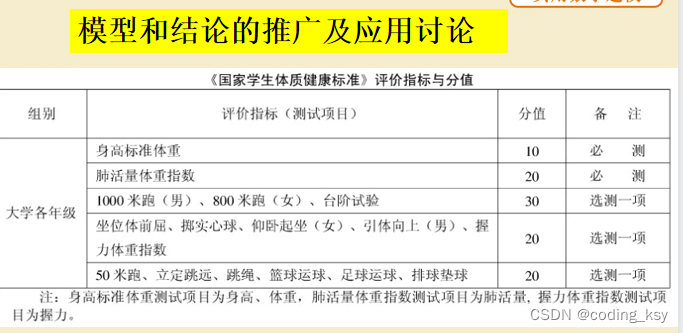### 力扣刷题---初始链表1

&#x1f388;个人主页:&#x1f388; :✨✨✨初阶牛✨✨✨ &#x1f43b;推荐专栏: &#x1f354;&#x1f35f;&#x1f32f; c语言初阶 &#x1f511;个人信条: &#x1f335;知行合一 &#x1f349;本篇简介:>:讲解初始数据结构链表的三个力扣题 1.移除链表元素. 2.反转…

### React面向组件编程（理解与使用+state+props+refs与事件处理）

1 基本理解与使用 函数式组件 <!DOCTYPE html> <html lang"en"> <head><meta charset"UTF-8"><meta http-equiv"X-UA-Compatible" content"IEedge"><meta name"viewport" content"…

### 最强分布式锁工具：Redisson

1 Redisson概述1.1 什么是Redisson&#xff1f;Redisson是一个在Redis的基础上实现的Java驻内存数据网格&#xff08;In-Memory Data Grid&#xff09;。它不仅提供了一系列的分布式的Java常用对象&#xff0c;还提供了许多分布式服务。其中包括(BitSet, Set, Multimap, Sorted…

### 讲解Linux中samba理论讲解及Linux共享访问

♥️作者&#xff1a;小刘在C站 ♥️个人主页&#xff1a;小刘主页 ♥️每天分享云计算网络运维课堂笔记&#xff0c;努力不一定有收获&#xff0c;但一定会有收获加油&#xff01;一起努力&#xff0c;共赴美好人生&#xff01; ♥️夕阳下&#xff0c;是最美的绽放&#xff0…